How Cheenta works to ensure student success?
Explore the Back-Story

# Combination of Cups | PRMO-2018 | Problem 11Try this beautiful problem from PRMO, 2018 based on Combination of Cups.

## Combination of Cups | PRMO | Problem 11

There are several tea cups in the kitchen, some with handle and the others without handles. The
number of ways of selecting two cups without a handle and three with a handle is exactly 1200.
What is the maximum possible number of cups in the kitchen ?

•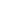••### Key Concepts

Algebra

combination

maximum

Answer:PRMO-2018, Problem 11

Pre College Mathematics

## Try with Hints

We assume that the number of cups with handle=m and number of cups without handle =n

Can you now finish the problem ..........

The way of select 3 cups with a handle from m cups =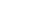and select 2 cups without a handle from n cups =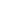Can you finish the problem........

We assume that the number of cups with handle=m and number of cups without handle =n

The way of select 3 cups with a handle from m cups =and select 2 cups without a handle from n cups =Therefore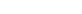=1200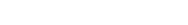=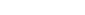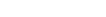and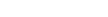Therefore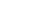be maximum i.e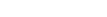Maximum possibile cups=25+4=29

## Subscribe to Cheenta at Youtube

Try this beautiful problem from PRMO, 2018 based on Combination of Cups.

## Combination of Cups | PRMO | Problem 11

There are several tea cups in the kitchen, some with handle and the others without handles. The
number of ways of selecting two cups without a handle and three with a handle is exactly 1200.
What is the maximum possible number of cups in the kitchen ?

•••### Key Concepts

Algebra

combination

maximum

Answer:PRMO-2018, Problem 11

Pre College Mathematics

## Try with Hints

We assume that the number of cups with handle=m and number of cups without handle =n

Can you now finish the problem ..........

The way of select 3 cups with a handle from m cups =and select 2 cups without a handle from n cups =Can you finish the problem........

We assume that the number of cups with handle=m and number of cups without handle =n

The way of select 3 cups with a handle from m cups =and select 2 cups without a handle from n cups =Therefore=1200=andThereforebe maximum i.eMaximum possibile cups=25+4=29

## Subscribe to Cheenta at Youtube

This site uses Akismet to reduce spam. Learn how your comment data is processed.

### Knowledge Partner